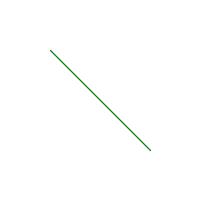# Wand line() function in Python

• Last Updated : 09 Feb, 2022

line() is another drawing function present in wand.drawing module. As the name implies line() function is used to draw a line in the image. line() function only need two arguments that are start and end point of the line that we want to draw.

Syntax :

`wand.drawing.line(start, end)`

Parameters :

Example #1:

## Python3

 `# Import required objects from wand modules``from` `wand.image ``import` `Image``from` `wand.drawing ``import` `Drawing``from` `wand.color ``import` `Color` `# generate object for wand.drawing``with Drawing() as draw:``    ``# set stroke color``    ``draw.stroke_color ``=` `Color(``'green'``)``    ``# set width for stroke``    ``draw.stroke_width ``=` `1``    ``draw.line(( ``50``, ``50``),  ``# Stating point``             ``( ``150``, ``150``))  ``# Ending point``    ``with Image(width ``=` `200``,``               ``height ``=` `200``,``               ``background ``=` `Color(``'white'``)) as img:``        ``# draw shape on image using draw() function``        ``draw.draw(img)``        ``img.save(filename ``=``'line.png'``)`

Output :Example #2: Draw a line on a pre-existing image.
Source Image:## Python3

 `# Import required objects from wand modules``from` `wand.image ``import` `Image``from` `wand.drawing ``import` `Drawing``from` `wand.color ``import` `Color` `# generate object for wand.drawing``with Drawing() as draw:``    ``# set stroke color``    ``draw.stroke_color ``=` `Color(``'white'``)` `    ``# set width for stroke``    ``draw.stroke_width ``=` `1``    ``with Image(filename ``=` `"gog.png"``) as img:``        ``draw.line((( img.height)``/``2``, ``0``),  ``# Stating point``                   ``( ``0``, (img.width)``/``2``))  ``# Ending point` `        ``# draw shape on image using draw() function``        ``draw.draw(img)``        ``img.save(filename ``=``'line2.png'``)`

Output :My Personal Notes arrow_drop_up